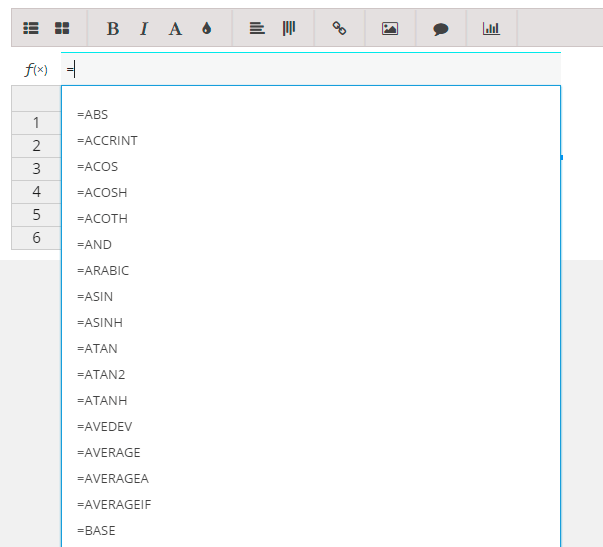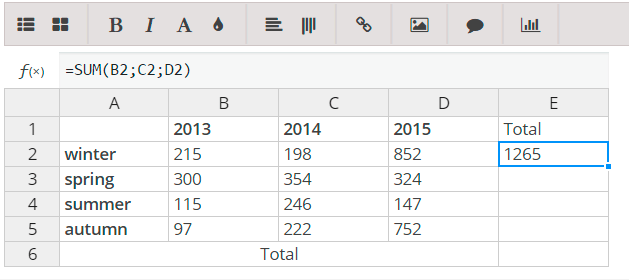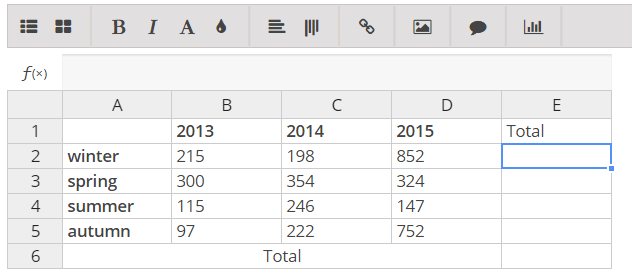﻿ Formulas in Table - Ultimate WordPress Plugins by Supsystic

# Formulas in Table

1. Go to Editor of Data Table.
2. Select a cell and start typing. In a cell, type an equal sign “=” to start the formula.

Check the list of formulas which supported by Data Tables on this page.1. Fill in the rest of the formula.
2. Do one of the following:

• Choose one of the available functions, then inside the parentheses “()” type the cells you need – inserting an operator between them. It will look like this `=SUM(B2;C2;D2)`
• Type a combination of numbers and operators; for example, `=3+7`.
• Type a name of cells (inserting an operator between them). For example, type B1 and then type a plus sign (+), type C1 and type +, and then type D1. `=B1+C1+D1`3. Save the changes of table.
4. Check the result of formula in the table on your site.

The result of the formula in the table you can’t see in the editor of table in WordPress admin area, only in the table on your site.

Categories
Latest Articles